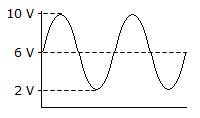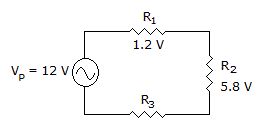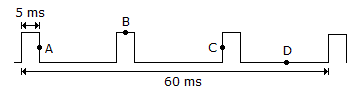# Electronics - Alternating Current and Voltage

### Exercise :: Alternating Current and Voltage - General Questions

11.What is the dc voltage of the waveform in the given circuit?

 A. 2 V B. 4 V C. 6 V D. 8 V

Answer: Option C

Explanation:

No answer description available for this question. Let us discuss.

12.

What is the fourth harmonic of a fundamental frequency of 400 Hz?

 A. 100 Hz B. 4 kHz C. 4 Hz D. 1.6 kHz

Answer: Option D

Explanation:

No answer description available for this question. Let us discuss.

13.What is the peak voltage across R3 in the given circuit?

 A. 1.2 V B. 5 V C. 7 V D. 12 V

Answer: Option B

Explanation:

No answer description available for this question. Let us discuss.

14.Which point is the trailing edge of the repetitive pulse waveform in the given circuit?

 A. Point A B. Point B C. Point C D. Point D

Answer: Option A

Explanation:

No answer description available for this question. Let us discuss.

15.

A single-phase induction motor

 A. is self-starting B. operates at a fixed speed C. is less reliable than a three-phase synchronous motor D. none of the above

Answer: Option D

Explanation:

No answer description available for this question. Let us discuss.

#### Current Affairs 2021

Interview Questions and Answers